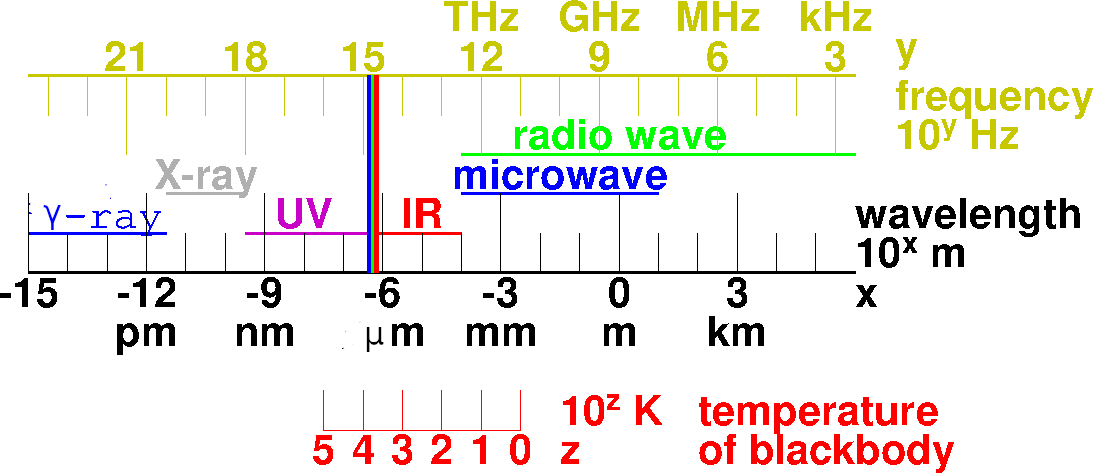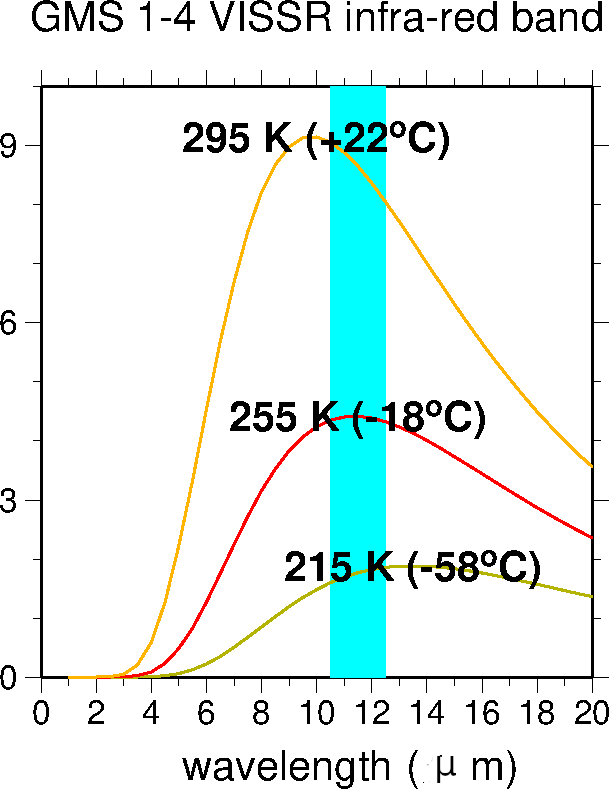﻿ Electromagnetic waves

Electromagnetic waves

Oscillation and waves

• Oscillation: variation of a certain quantity, repeating at a constant time interval.
• Period: the time interval between the same state. SI unit: second [s].
• Frequency: the reciprocal of "period". SI unit: 1/s = hertz [Hz].
• [Note] The words "period" and "frequency" have special meanings with oscillation and waves.
• Waves: oscillation propagating in space. (Not motion of material bodies.)
• Wavelength: the length from a peak to the next peak. SI unit: metre [m].
• Wavenumber: the reciprocal of "wavelength". SI unit: 1/m .
• Speed of wave = wavelength / period = wavelength × frequency. SI Unit: m/s.

Wavelength and frequency of electromagnetic waves• Electromagnetic waves are such waves that the variation of electric fields and that of magnetic fields are conbined.
• λ ν = c ... where λ:wavelength; ν[nu]:frequency; c: speed of light.
• The speed of light in vacuum is one of the fundamental constants of physics. The value is approximately "7.5 times around the earth" per second.
• The speed of light in air varies with temperature, water vapor content, etc. But it can be regarded as constant approximately.
• The path of light in vacuum is a straight line according to classical physics. (It is curved by gravity according to the general theory of relativity. But, classical physics gives good approximation for everyday life.)
• Electromagnetic waves are also particles called photons. Energy is transmitted in a lump of h ν, where h is Planck's constant, and ν is frequency.
• Therefore, electromagnetic waves of shorter wavelength can give stronger damages to chemical compounds (including such molecules as DNA that are essential to living organisms).

Interaction of radiation (= electromagnetic waves, photons) and material bodies

• emission ... Photons appear. Energy of the material body decreases.
• absorption ... Photons disappear. Energy of the material body increases.
• scattering ... Direction of photon's motion is changed. Energy of the material body remains the same.

Radiative transfer of energy across a layer of space

• electomagnetic waves that have arrived at the layer can be:
• absorbed ... energy of the matter in the layer increses.
• reflected (or scattered backwards)
• transmitted (or scattered forewards)
• electromagnetic waves emitted by the layer (to both sides) ... energy of the matter in the layer decreases.

Radiative transfer at an opaque surface

• electromagnetic waves arrived at the layer ... α[reflectivity or "albedo"] reflected, (1-α) absorbed
• electromagnetic waves emitted by the layer ... ε[emissivity] × blackbody radiation
• absorptivity (1-α) = emissivity ε [Kirchhoff's Law on radiation], at each wavelength

• Every material body emits radiation according to its temperature and emissivity.
• "Blackbody" is an ideal body whose emissivity is 1 in all wavelengths. Its absorptivity is also 1 in all wavelengths, so it is ideally black.
• Thermal radiation of a real body is blackbody radiation multiplied by (wavelength-dependent) emissivity of the body.
• Energy flow of blackbody radiation per unit area of surface per unit time is proportional to T4, where T is (absolute) temperature. (Stefan-Boltzmann Law). The coefficient of proportionality is called Stefan-Boltzmann constant (σB).

Energy spectrum (distribution of energy with respect to wavelength) of blackbody radiationNote: logarithmic scale in both axis

Peak wavelenth is inversely proportional to temperature. (Wien's Law)Note: logarithmic scale in both axisNote: linear (not logarithmic) scale in both axis

2019-May-16
MASUDA Kooiti